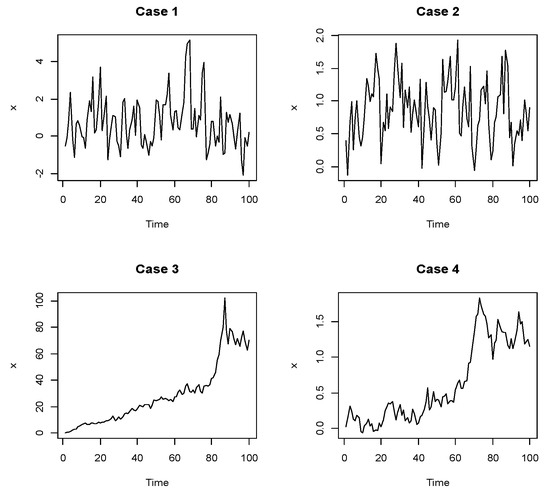Previous Article in Journal
Quasi (s,r)-Contractive Multi-Valued Operators and Related Fixed Point Theorems
Open AccessArticle

# Comparing Parameter Estimation of Random Coefficient Autoregressive Model by Frequentist Method

byAutcha Araveeporn
Department of Statistics, Faculty of Science, King Mongkut’s Institute of Technology Ladkrabang, Bangkok 10520, Thailand
Mathematics 2020, 8(1), 62; https://doi.org/10.3390/math8010062
Received: 20 November 2019 / Revised: 20 December 2019 / Accepted: 20 December 2019 / Published: 2 January 2020
This paper compares the frequentist method that consisted of the least-squares method and the maximum likelihood method for estimating an unknown parameter on the Random Coefficient Autoregressive (RCA) model. The frequentist methods depend on the likelihood function that draws a conclusion from observed data by emphasizing the frequency or proportion of the data namely least squares and maximum likelihood methods. The method of least squares is often used to estimate the parameter of the frequentist method. The minimum of the sum of squared residuals is found by setting the gradient to zero. The maximum likelihood method carries out the observed data to estimate the parameter of a probability distribution by maximizing a likelihood function under the statistical model, while this estimator is obtained by a differential parameter of the likelihood function. The efficiency of two methods is considered by average mean square error for simulation data, and mean square error for actual data. For simulation data, the data are generated at only the first-order models of the RCA model. The results have shown that the least-squares method performs better than the maximum likelihood. The average mean square error of the least-squares method shows the minimum values in all cases that indicated their performance. Finally, these methods are applied to the actual data. The series of monthly averages of the Stock Exchange of Thailand (SET) index and daily volume of the exchange rate of Baht/Dollar are considered to estimate and forecast based on the RCA model. The result shows that the least-squares method outperforms the maximum likelihood method. View Full-Text
Show FiguresFigure 1

MDPI and ACS Style

Araveeporn, A. Comparing Parameter Estimation of Random Coefficient Autoregressive Model by Frequentist Method. Mathematics 2020, 8, 62.